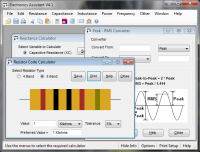# Capacitance Unit Converter

The calculator will convert between units commonly used for capacitance. Enter the value to convert, and the units to convert from and to. The converted value will be shown on the right. A conversion table showing all the values can be found here in the data archive.

#### Convert Between Units:

 Convert Value: From (Units): Farads Millifarads Microfarads Nanofarads Picofarads To (Units): Farads Millifarads Microfarads Nanofarads Picofarads Value = ?

# If you found this calulator useful you should try Electronics Assistant!• All the online calculators and more in a stand-alone application
• Converts Resistor & Inductor colour codes, calculates LED series resistors, capacitance units, series / parallel resistors & capacitors, frequency, reactance & more
• Calculation of nearest preferred resistor values with a choice of 5 series from E12 to E192
• Print & save calculation results